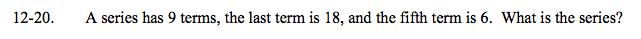### Home > A2C > Chapter 12 > Lesson 12.1.1 > Problem12-20

12-20.Find the sequence that corresponds to the terms of the series.

t(5) = 6 = (5)a + b
t(9) = 18 = (9)a + b

a = 3 and b = −9

Now use the sequence to find the rest of the terms of the series.

(−6) + (−3) + 0 + 3 + 6 + 9 + 12 + 15 + 18